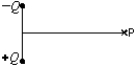Electric Fields

# Fundamentals of Physics Study Set 2

Physics & Astronomy

## Quiz 22 :Electric FieldsStudy FlashcardsLooking for Introductory Physics Homework Help?## Quiz 22 :Electric Fields

Showing 1 - 20 of 52The units of the electric field are:
Free
Multiple Choice

DThe units of the electric field are:
Free
Multiple Choice

AAn electric field is most directly related to:
Free
Multiple Choice

DAs used in the definition of electric field, a "test charge":
Multiple ChoiceExperimenter A uses a test charge q0 and experimenter B uses a test charge 2q0 to measure an electric field produced by stationary charges.A finds a field that is:
Multiple ChoiceElectric field lines:
Multiple ChoiceTwo thin spherical shells, one with radius R and the other with radius 2R, surround an isolated charge point particle.The ratio of the number of field lines through the larger sphere to the number through the smaller is:
Multiple ChoiceA certain physics textbook shows a region of space in which two electric field lines cross each other.We conclude that:
Multiple ChoiceChoose the correct statement concerning electric field lines:
Multiple ChoiceThe diagram shows the electric field lines due to two charged parallel metal plates.We conclude that: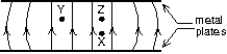Multiple ChoiceThe diagram shows the electric field lines in a region of space containing two small charged spheres (Y and Z).Then: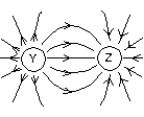Multiple ChoiceTwo protons (p1 and p2)are on the x axis, as shown below.The directions of the electric field at points 1, 2, and 3 respectively, are: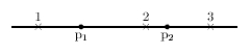Multiple ChoiceLet k denote 1/40.The magnitude of the electric field at a distance r from an isolated point charge q is:
Multiple ChoiceThe electric field at a distance of 10 cm from an isolated point particle with a charge of 2 10-9 C is:
Multiple ChoiceAn isolated point charged point particle produces an electric field with magnitude E at a point 2 m away from the charge.A point at which the field magnitude is E/4 is:
Multiple ChoiceAn isolated charged point particle produces an electric field with magnitude E at a point 2 m away.At a point 1 m from the particle the magnitude of the field is:
Multiple ChoiceThe equation E = kq/r2 applies to:
Multiple ChoiceTwo point particles, with charges of q1 and q2, are placed a distance r apart.The electric field is zero at a point P between the particles on the line segment connecting them.We conclude that:
Multiple ChoiceThe diagrams below depict four different charge distributions.The charged particles are all the same distance from the origin.The electric field at the origin: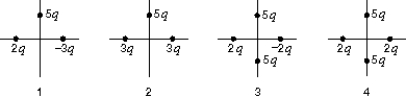The diagram shows a particle with positive charge Q and a particle with negative charge Q.The electric field at point P on the perpendicular bisector of the line joining them is: